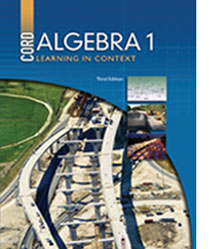# Algebra 1 - 3rd Edition

## Chapter 6: Probability

Some links are repeated for use with more than one lesson.

### This site applies to the entire chapter.

http://www.explorelearning.com/index.cfm?method=cResource.dspResourcesForCourse&CourseID=268
This site contains gizmos that demonstrate different probability simulations.

http://education.ti.com/xchange/US/Math/Statistics/9327/ProbabilitySimulations_Student.pdf
A short PDF handout demonstrating how to use the TI-8x family of calculators to generate and tally random numbers, simulating probability experiments.

### 6.1 Probability

http://www.freemathhelp.com/Lessons/PreAlgebra_Probability_BB.htm
This site contains a video tutorial with notes covering an introduction to probability.

http://www.mathgoodies.com/lessons/vol6/intro_probability.html
This page contains an explanation on probability with interactive examples of probability experiments including dice and a spinner.

http://mathforum.org/dr.math/faq/faq.prob.intro.html

### 6.2 Experimental Probability

http://education.ti.com/en/us/software/details/en/35444E80EDC34A0CBCD17F3C5EFA0A99/83probabilitysimulation Probability simulation application for the TI-83/84 family of calculators. (Can download as “guest.”)

http://www.shodor.org/interactivate/activities/ExpProbability/
An introduction to experimental probability, and several interactive activities to demonstrate it. This website requires Java to run some of its features.

### 6.3 The Addition Principle of Counting

http://www.tutorvista.com/content/math/discrete-math/permutations-and-combinations/fundamental-principle-counting.php

### 6.4 The Fundamental Counting Principle

This site contains a basic definition of the fundamental counting principle and an example.

http://www.algebra-class.com/fundamental-counting-principle.html
NEW Short tutorial on the fundamental counting principle, or "sample space,", and using it to calculate simple probability.

### 6.5 Independent and Dependent Events

http://www.learningwave.com/chapters/probability/dependent_independent.html